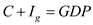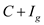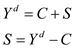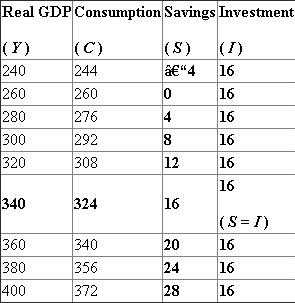# Quiz 28: Basic Macroeconomic Relationships

Equilibrium real GDP occurs whenin a private economy because it is the level of output at which the total quantity of goods produced (or the GDP) equals the total quantity of goods purchased (). Ifwere to exceed GDP, then that means that the spending in the economy is higher than the production of the economy. Excess spending in the economy will bring down inventories of goods and thus encourage firms to increase production. Firms will increase production (so GDP will increase) until, where there is no overproduction or excess total spending. On the other hand if, is less than GDP, then this means that there is overproduction in the economy. Sincerepresents the spending in the economy, the production of the economy is higher than what is being spent, meaning that there will be inventory buildups, which causes firms to not want to produce as much. Thus, firms will decrease production, which decreases GDP. GDP will decrease until the equilibriumis reached, where there is no overproduction or excess total spending. The two expenditure components of real GDP that are excluded in a private closed economy are government spending and net exports. Because the economy is private, there is no government, and because the economy is closed, there is no trade.
(a) First, remember that(consumption plus gross investment equals GDP). A decline in the real interest rate will cause investment spending to increase. When the real interest rate falls, this means that businesses who want to borrow money to finance investment projects will find it cheaper to borrow that money. A lower interest rate implies that total amount of money a business would need to return in the future is lower, and thus the business may find it more worthwhile to borrow money and spend it on an investment project. A decline in real interest rate will also cause consumption to go up, as household can now borrow at a cheaper rate. Households would then want to borrow more and consume more, thus increasing consumption. Since both consumption and investment spending increases, a decline in real interest rate will increase real GDP. (b) If there is an overall decrease in the expected rate of return on investment, then this means that businesses will be less likely wanting to invest. A lower expected rate of return on investment means that any investment project a firm wants to undertake will have a lower return. Thus, some projects may no longer be profitable to start. Because of this, investment spending will fall as firms invest less, which will cause GDP to decrease. (c) A sizable, sustained increase in stock prices will cause household wealth to increase. In other words, consumers will feel like they are richer because they have more wealth. Having more wealth is associated with higher consumption and lower saving, thus we see that in the economy, consumption will increase. Since GDP is equal to consumption plus investment, we see that GDP will increase (since investment does not decrease).
Marginal propensity to consume (MPC) measures the degree of responsiveness of a change in the consumption spending due to a given change in the income of a consumer. That is, it is the additional amount spent on consumption due to an increase in the income by an additional dollar. For this reason, MPC always lies between 0 anD1. Marginal propensity to save (MPS) measures the degree of responsiveness of a change in the savings of a consumer for a given change in his/her income level. That is, it is the additional number of dollars that is saved, when the income of a consumer increases by one additional dollar. Disposable income ( Y ) consists of consumption ( C ) and saving ( S ). The following relationship holds between these components:The following table shows the level of savings at each GDP level (figures are in billions):The equilibrium occurs at the point of intersection of savings and investment curves. That is, both savings and investment are equal at equilibrium. Here, savings and investment are equal (\$16 billion) at an equilibrium level of output of \$340 billion. The equilibrium employment level is '65' million. The value of MPC is calculated as follows:Thus, the value of MPC is 0.8. This means that the consumer spends 80 cents out of an additional dollar he/she earns. The value of MPS is calculated as follows:Hence, the value of MPS is 0.2. This means that the consumer saveS20 cents out of an additional dollar he/she earns.